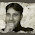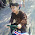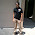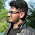# How to Check if Given Number is Prime in Java - With Example

One of the most common programming exercise for beginners is, write a program to check if given number is prime or not? There are many methods to check if a number is prime or not, but most common of them is trial division, which is what we will see in this tutorial. In my opinion, these kind of program is their first steps towards algorithmic understanding. You first come with a solution, which is driven by the fact that prime numbers are natural numbers, which are not divisible by any positive number other than 1 and themselves. You write a for loop to check every number, starting from 1 to given number, to see if given number is divisible by any positive number or not. This leads you to the solution. Then you find some more fact that there is no need to check till N-1, where N is the number we are checking for primeness, and checking till square root of N is enough. This reduces a lot of time, especially while checking a large number is prime or not.

Further, you come to know that if its not divisible by 2 then there is no need to checking for any other even number, and you increment counter by 2, instead of 1. So in a way, you learn how to optimize your solution by taking advantage of facts available.

After this you can try something like Fibonacci series, or may be finding factorial of a number in Java to do some more practice on programming. This will not only teach you language basics e.g. loops, control statements like if-else, use of arithmetic and relational operator but also helps to develop programming logic.

By the way, you can even take this problem of checking if a number is prime or not, to next level by try to implement different algorithms for finding primes e.g. sieve of Atkin or  sieve of Eratosthenes. In fact in programming challenges, you often need to build your prime number cache up-to a certain number to progress further in finding solution.

This question is also asked on written test and interview as  how to print prime numbers from 1 to 100  or finding prime factor of a number in Java.

## Java Program to Check if an Integer is Prime or Not

This is our Java program to see if a given integer number is prime or not. As I said, a number is called a prime number if its only divisible by 1 or itself, which means prime number doesn't have any positive divisor other than itself. There are many ways to check if number is prime or not, or generating list of primes. Easiest of them is known as trial division, which is a natural way of finding prime. In trial division, you divide . It consists of testing whether n is a multiple of any integer between 2 and sqrt{n}.  In this program I have presented three solution or method to check if number is prime. First solution is implementation of trial division, where we are checking from 2 to sqrt(n), we are using java.lang.Math class for calculating square root. Since this function returns double, we need to cast result back into integer. Our second method of checking if a number is prime or not is little bit optimized that this as it doesn't check division by even numbers other than two.Third method is most optimized of all three methods of prime number checking. By the way there is another exercise for you to do after this is checking if a number is  Armstrong number  or not.

```import java.util.Scanner;
/**
* Java Program to check if a number is Prime or Not. This program accepts a
* number from command prompt and check if it is prime or not.
*
* @author  http://java67.blogspot.com
*/
public class Testing {

public static void main(String args[]) {
Scanner scnr = new Scanner(System.in);
int number = Integer.MAX_VALUE;
System.out.println("Enter number to check if prime or not ");
while (number != 0) {
number = scnr.nextInt();
System.out.printf("Does %d is prime? %s %s  %s %n", number,
isPrime(number), isPrimeOrNot(number), isPrimeNumber(number));
}
}

/*
* Java method to check if an integer number is prime or not.
* @return true if number is prime, else false
*/
public static boolean isPrime(int number) {
int sqrt = (int) Math.sqrt(number) + 1;
for (int i = 2; i < sqrt; i++) {
if (number % i == 0) {
// number is perfectly divisible - no prime
return false;
}
}
return true;
}

/*
* Second version of isPrimeNumber method, with improvement like not
* checking for division by even number, if its not divisible by 2.
*/
public static boolean isPrimeNumber(int number) {
if (number == 2 || number == 3) {
return true;
}
if (number % 2 == 0) {
return false;
}
int sqrt = (int) Math.sqrt(number) + 1;
for (int i = 3; i < sqrt; i += 2) {
if (number % i == 0) {
return false;
}
}
return true;
}

/*
* Third way to check if a number is prime or not.
*/
public static String isPrimeOrNot(int num) {
if (num < 0) {
return "not valid";
}
if (num == 0 || num == 1) {
return "not prime";
}
if (num == 2 || num == 3) {
return "prime number";
}
if ((num * num - 1) % 24 == 0) {
return "prime";
} else {
return "not prime";
}
}
}

Output
Enter number to check if prime or not
2? Does 2 is prime? true prime number  true
3? Does 3 is prime? true prime number  true
4? Does 4 is prime? false not prime  false
5? Does 5 is prime? true prime  true
6? Does 6 is prime? false not prime  false
7? Does 7 is prime? true prime  true
17? Does 17 is prime? true prime  true
21? Does 21 is prime? false not prime  false
131? Does 131 is prime? true prime  true
139? Does 139 is prime? true prime  true```

That's all in this program about how to check if a number is prime or not. number must be integer, as concept of prime is only for natural numbers and not for floating point numbers. As I said there are couple of more algorithms for checking if a number is prime or not, and some of the algorithm is optimized for finding prime numbers. It imperative to know at-least one fast way of finding prime number,as this trial division is not fast enough for real world problems. I suggest exploring sieve of Atkin and sieve of Eratosthenes way of finding prime numbers.

Further Learning
The Coding Interview Bootcamp: Algorithms + Data Structures
Data Structures and Algorithms: Deep Dive Using Java
Algorithms and Data Structures - Part 1 and 2

#### 28 comments:

1.Your isPrimeOrNot() method is not a correct way to detemine if a number is prime. (num * num - 1 ) % 24 == 0 is a property that is true for all primes but it can be true for some non primes as well eg num = 25.

Also I think the code would clearer if you didn't add one to sqrt but instead used i <= sqrt in your for() loop.

1.int sqrt = (int) Math.sqrt(number) + 1;
for (int i = 3; i < sqrt; i += 2) {
if (number % i == 0) { return false;
}
}

This is a flawed approach. It counts 15 as a prime, where 5 is not (15 % 3 = 0).

2.The code is not valid to check the number is prime or not, which is written in the method isPrimeOrNot(int num).
This method will also print some non-prime number like: 25,35,49...

1.You are correct the code has bug, it prints 25 has prime number.

3.Check this out.
I think its the best way to do without any errors or bugs.
beginners may also like it as it lucid and easy.

int limit = 100;

System.out.println("Prime numbers between 1 and " + limit);

//loop through the numbers one by one
for(int i=1; i < 100; i++){

boolean isPrime = true;

//check to see if the number is prime
for(int j=2; j < i ; j++){

if(i % j == 0){
isPrime = false;
break;
}
}
// print the number
if(isPrime)
System.out.print(i + " ");
}

Ashim Kumar Halder
Android Developer
ashimkr.halder@gmail.com

1.Thanks alot

4.You should add line if(number < 2) return false; to method isPrimeNumber

1.Hi, actually if(number %2==0) will also check number<2 condition.
1%2 equals 0 or -4%2 equals 0.

5.6.public static boolean isPrime(int number){
if(number%2==0){
return false;
}
for(int i=3;i<number;i=i+2){
if(number%i==0){
return false;
}
}
return true;
}

7.hi I hope this works

import java.util.Scanner;

public class Prime {
public static void main(String[] args) {
Prime p= new Prime();
Scanner sc= new Scanner(System.in);
System.out.println("Enter the number to check its prime or not: ");
int num=sc.nextInt();
System.out.println(p.primenum(num));
}
private boolean primenum(int num)
{
boolean isprime=true;
if(num==2||num==3)
{
return isprime;
}
if(num%2==0)
{
return isprime=false;
}
for(int i=3;i<num;i+=2)
{
if(num%i==0)
{
return isprime=false;
}
}
return isprime;

}

}

8.Hi, this program checks if a number (+ve integer) entered is prime or not. It prompts the user to enter a +ve integer, checks it and displays if it's a prime number or not.

Try and run the program. If there is any question or comment on the program, please drop it here. Thanks
..............................................................................

import java.util.Scanner ;

public class PrimeNumbers
{
public static void main(String[] args)
{
Scanner input = new Scanner(System.in) ;

int counter = 1;

System.out.printf(" \n Please enter a +ve Integer :") ;
int num = input.nextInt() ;

if (num == 1 || num % num == 0)
{
++counter ;

if (num == 1)
{
--counter ;
}
}

for (int i = 2; i <= num; i++)
{
if (( num == i ) ^ (num % i == 0))
{
counter ++;
}
}

if ( counter == 2)
{
System.out.printf("\n %d is a prime number", num) ;
}
else
{
System.out.printf("\n %d is not a prime number", num) ;
}
}
}

1.Can you explain the logic? Why you are checking counter and what is the use of bitwise ^ operator here?

9.import java.util.Scanner ;

public class PrimeNumbers
{
public static void main(String[] args)
{
int i,count=1;
Scanner input = new Scanner(System.in) ;
System.out.printf(" \n Please enter an Integer :") ;
int num = input.nextInt() ;
for(i=2;i0)
{
System.out.printf("\n %d is a prime number", num) ;
}
else
{
System.out.printf("\n %d is not a prime number", num) ;
}
}
}

1.import java.util.Scanner ;

public class PrimeNumbers
{
public static void main(String[] args)
{
int i,count=1;
Scanner input = new Scanner(System.in) ;
System.out.printf(" \n Please enter an +op Integer :") ;
int num = input.nextInt() ;
for(i=2;i0)
{
System.out.printf("\n %d is a prime number", num) ;
}
else
{
System.out.printf("\n %d is not a prime number", num) ;
}
}
}

2.import java.util.Scanner;
public class Main{

public static void main(String []args)
{
int a;
boolean flag=false;
System.out.println("enter number:");
Scanner sc=new Scanner(System.in);
a=sc.nextInt();
for(int i=2;i<=a/2;i++)
{
if(a%i==0)
{
flag=true;
break;
}
}
if(!flag)
System.out.println("is prime");
else
System.out.println("is not prime");
}

}

10.public static boolean isPrime(int num) {
boolean isPrime = true;
for(int i = 2 ; i <=num/2; i++) {
if(num%i==0) {
isPrime = false;
break;
}
}
return isPrime;
}

11.public static boolean isPrime(int num) {
boolean isPrime = true;
for(int i = 2 ; i <=num/2; i++) {
if(num%i==0) {
isPrime = false;
break;
}
}
return isPrime;
}

12.public class PrimeExample{
public static void main(String args[]){
int i,m=0,flag=0;
int n=3;//it is the number to be checked
m=n/2;
if(n==0||n==1){
System.out.println(n+" is not prime number");
}else{
for(i=2;i<=m;i++){
if(n%i==0){
System.out.println(n+" is not prime number");
flag=1;
break;
}
}
if(flag==0) { System.out.println(n+" is prime number"); }
}//end of else
}
}

1.public class PrimeExample2{
static void checkPrime(int n){
int i,m=0,flag=0;
m=n/2;
if(n==0||n==1){
System.out.println(n+" is not prime number");
}else{
for(i=2;i<=m;i++){
if(n%i==0){
System.out.println(n+" is not prime number");
flag=1;
break;
}
}
if(flag==0) { System.out.println(n+" is prime number"); }
}//end of else
}
public static void main(String args[]){
checkPrime(1);
checkPrime(3);
checkPrime(17);
checkPrime(20);
checkPrime(53);
checkPrime(1289);
checkPrime(2017);
checkPrime(6933);
}
}

13.public class PrimeExample{
public static void main(String args[]){
int i,m=0,flag=0;
int n=3;//it is the number to be checked
m=n/2;
if(n==0||n==1){
System.out.println(n+" is not prime number");
}else{
for(i=2;i<=m;i++){
if(n%i==0){
System.out.println(n+" is not prime number");
flag=1;
break;
}
}
if(flag==0) { System.out.println(n+" is prime number"); }
}//end of else
}
}

14.public class CheckPrimeNumber {

public static void main(String[] args) {

int temp;
boolean isPrime= true;
Scanner s = new Scanner(System.in);
int number = Integer.MAX_VALUE;
System.out.println("Enter a number to check prime:");
number = s.nextInt();
s.close();

for(int i=2;i<=number/2;i++)
{
temp= number%i;
if(temp==0)
{
isPrime = false;
break;
}
}
if(isPrime)
System.out.println(number+"is a prime number");
else
System.out.println(number+"is not a prime number");

}

}

15.why are we adding 1 to sqrt of number?

16.import java.util.*;
import java.io.*;
import java.math.*;

class Prime
{
public static void main(String args[])
{
Scanner sc=new Scanner(System.in);
int i,n,j,k=0;

System.out.println("Enter any Number:");
n=sc.nextInt();

j=(int)Math.sqrt(n);

for(i=2;i<=j;i++)
{
if(n%i==0)
{
k++;
}
else
;
}
if(k==0)
System.out.println("Prime Number");
else
System.out.println("Not a Prime Number");
}
}

17.hi,
please verify whether it is correct:

public class task {

public static void main(String[] args) {
int a=238;
if(((a%2==0)||(a%3==0))&&((a!=2)||(a!=3))) {
System.out.print("given num is not prime");
}
else {
System.out.print("given num is prime");
}
}

}

18.please verify whether it is valid:

public class task {

public static void main(String[] args) {
int a=238;
if(((a%2==0)||(a%3==0))&&((a!=2)||(a!=3))) {
System.out.print("given num is not prime");
}
else {
System.out.print("given num is prime");
}
}

}

19.public class Test {

public static void main(String[] args) {
int number = 111;
for(int i=2;i<Math.sqrt(number);i++) {
if(number%i==0) {
System.out.println("nt prime number" + i);
return;
}
}
System.out.println(" prime");
}
}

20.int sqrt = (int) Math.sqrt(number) + 1;
for (int i = 3; i < sqrt; i += 2) {
if (number % i == 0) {
return false;
}
}

This is flawed. 15 returns as prime. 15 % 3 is 0. I made an if statement, that could be implemented as a switch, that checks the number if it is divisible by the first 4 natural prime numbers (2, 3, 5, 7).